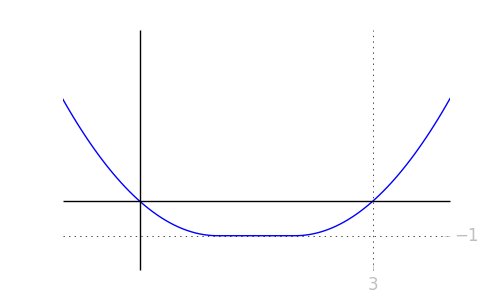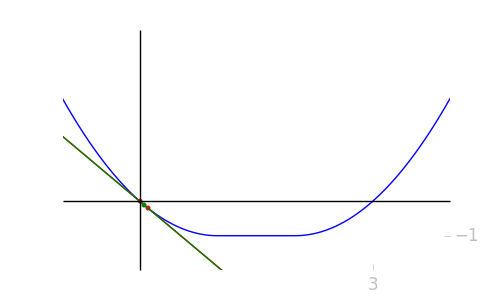Continuity of the Mean Value

1. Introduction

When I first learned the mean value theorem as a high schooler, I was thoroughly unimpressed. Part of this was because it’s just like Rolle’s Theorem, which feels obvious. But I think the greater part is because I thought it was useless. And I continued to think it was useless until I began my first proof-oriented treatment of calculus as a second year at Georgia Tech. Somehow, in the interceding years, I learned to value intuition and simple statements.

I have since completely changed my view on the mean value theorem. I now consider essentially all of one variable calculus to be the Mean Value Theorem, perhaps in various forms or disguises. In my earlier note An Intuitive Introduction to Calculus, we state and prove the Mean Value Theorem, and then show that we can prove the Fundamental Theorem of Calculus with the Mean Value Theorem and the Intermediate Value Theorem (which also felt silly to me as a high schooler, but which is not silly).

In this brief note, I want to consider one small aspect of the Mean Value Theorem: can the “mean value” be chosen continuously as a function of the endpoints? To state this more clearly, first recall the theorem:

Suppose ${f}$ is a differentiable real-valued function on an interval ${[a,b]}$. Then there exists a point ${c}$ between ${a}$ and ${b}$ such that $$\frac{f(b) – f(a)}{b – a} = f'(c), \tag{1}$$
which is to say that there is a point where the slope of ${f}$ is the same as the average slope from ${a}$ to ${b}$.

What if we allow the interval to vary? Suppose we are interested in a differentiable function ${f}$ on intervals of the form ${[0,b]}$, and we let ${b}$ vary. Then for each choice of ${b}$, the mean value theorem tells us that there exists ${c_b}$ such that $$\frac{f(b) – f(0)}{b} = f'(c_b).$$
Then the question we consider today is, as a function of ${b}$, can ${c_b}$ be chosen continuously? We will see that we cannot, and we’ll see explicit counterexamples. This, after the fold.

2. A Counterexample

For ease, we will restrict ourselves to intervals of the form ${[0,b]}$, as mentioned above. A particularly easy counterexample is given by $$f(x) = \begin{cases} x^2 – 2x & x \leq 1\\ -1 & -1 \leq x \leq 2\\ x^2 – 4x + 3 & x \geq 2 \end{cases}$$
This is a flattened parabola, that is, a parabola with a flattened middle section.Clearly, the slope of the function ${f}$ is negative until ${x = 1}$, where it is ${0}$. It becomes (and stays) positive at ${x = 2}$. So if you consider intervals ${[0,b]}$ as ${b}$ is varying, since ${f(b) < 0}$ for ${x < 3}$, we must have that ${c_b}$ is at a point when ${f'(c_b) < 0}$, meaning that ${c_b \in [0, 1]}$. But as soon as ${b > 3}$, ${f(b) > 0}$ and ${c_b}$ must be a point with ${f'(c_b) > 0}$, meaning that ${c_b \in [2,b]}$.

In particular, ${c_b}$ jumps from at most ${1}$ to at least ${2}$ as ${b}$ goes to the left of ${3}$ to the right of ${3}$. So there is no way to choose the ${c_b}$ values locally in a neighborhood of ${3}$ to make the mean values continuous there.

In the gif below, we have animated the process. The red line is the secant line passing through ${(0, f(0))}$ and ${(b, f(b))}$. The two red dots indicate the two points of intersection. The green line is the line guaranteed by the mean value theorem. The small green dot is, in particular, what we’ve been calling ${c_b}$: the guaranteed mean value. Notice that it jumps when the red dot passes ${3}$. That is the essence of this proof.Further, although this example is not smooth, it is easy to see that if we “smoothed” off the connection between the parabola and the straight line, like through the use of bump functions, then the spirit of this counterexample works, and not even smooth functions have locally continuous choices of the mean value.

This post appears on the author’s personal website davidlowryduda.com. It is also available in pdf note form. It was typeset in \TeX, hosted on WordPress sites, converted using the utility github.com/davidlowryduda/mse2wp, and displayed with MathJax. As usual, if there are any comments or questions, please let me know.

This entry was posted in Expository, Mathematics and tagged , , , , . Bookmark the permalink.